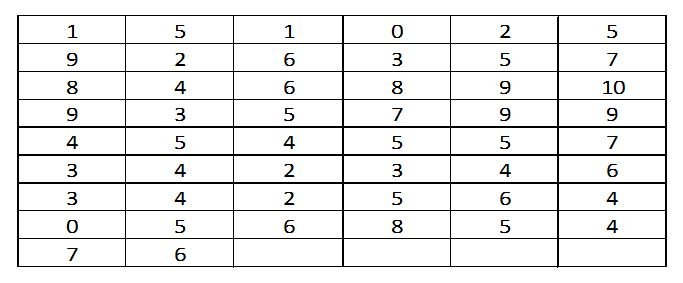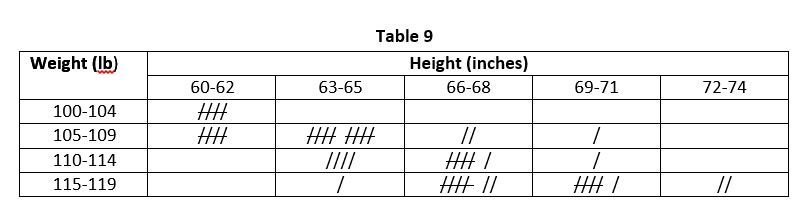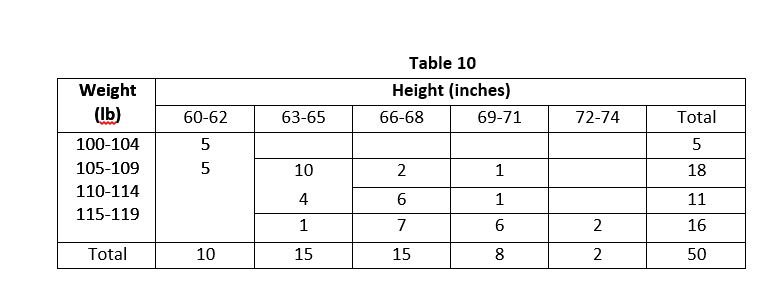Select Page

## Introduction

Frequency distribution table can be presented in various ways, depending upon the usage and utilization of the data. Frequency distribution is divided into several kinds also due to nature of raw data. Much useful information can be inferred from the frequency distribution table; therefore, frequency distribution table can be presented in proper and useful manner. Following are the various types of frequency distribution;

## 1. Frequency Distribution for Discrete Data

The class limits in discrete data are the true class limits and there will be no class boundaries because discrete data are not in fractions. For example; following figures represents number of children born to 50 women in a certain locality up to the age of 40 years.Are you interested in creating an Academic Blog just like econtutorials.com? Check my detailed step-by-step tutorials of creating your own blog in 2018.

The following Table 5 shows the frequency distribution table for discrete data, taking the class interval size of 1.

 Number of Children Tally Marks Number of Women 0 // 2 1 // 2 2 //// 4 3 //// 5 4 //// /// 8 5 //// //// 10 6 //// / 6 7 //// 4 8 /// 3 9 //// 5 10 / 1 Total 50

## 2. Cumulative Frequency Distribution

Cumulative frequency distribution represents the sum of all succeeding or previous frequencies up to certain class. The table showing the cumulative frequency is called cumulative frequency distribution or cumulative frequency distribution table or simply cumulative frequency. For example, referring Table 1, the cumulative frequency for class 120-129 is 1 + 4 = 5. Similarly, the cumulative frequency of the class 130-139 is 1+ 4 + 17 = 22. It will be interpreted as there are 22 children who have weights less than 139.5 pounds.

The cumulative frequency is shown in the following Table 6.

 Weights (lb) Cumulative Frequency Less than 109.5 0 Less than 119.5 0 + 1 = 1 Less than 129.5 1 + 4 = 5 Less than 139.5 5 + 17 = 22 Less than 149.5 22 + 28 = 50 Less than 159.5 50 + 25 = 75 Less than 169.5 75 + 18 = 93 Less than 179.5 93 + 13 = 106 Less than 189.5 106 + 6 = 112 Less than 199.5 122 + 5 = 117 Less than 209.5 117 + 2 = 119 Less than 219.5 119 + 1 = 120

## 3. Relative Frequency Distribution

The frequency of a class divided by the total frequency is called the relative frequency of that particular class. The frequency distribution table showing the relative frequencies is called relative frequency distribution or relative frequency or percentage table. Relative frequencies are generally expressed as a percentage. The sum of the relative frequencies of all the classes is 1 or 100%. For example; referring Table 1, the relative frequency of the class 160-169 is 18/120 x 100 = 15%. The following t Table 7 gives the relative frequency distribution for the weight distribution of Table 1.

 Weight (lb) Relative Frequency 110-119 1/120 = 0.0083 or 0.83% 120-129 4/120 = 0.0333 or 3.33% 130-139 17/120 = 0.1417 or 14.17% 140-149 28/120 = 0.2333 or 23.33% 150-159 25/120 = 0.2084 or 20.84% 160-169 18/120 = 0.15 or 15% 170-179 13/120 = 0.1083 or 10.83% 180-189 6/120 = 0,05 or 5% 190-199 5/120 = 0.0417 or 4.17% 200-209 2/120 = 0.0167 or 1.67% 210-219 1/120 = 0.0083 or 0.83%

## 4. Relative Cumulative Frequency Distribution

The cumulative frequency of a class divided by the total frequency is called relative cumulative frequency. It is also called percentage cumulative frequency since it is expressed in percentage. The table showing relative cumulative frequencies is called the relative cumulative frequency distribution or percentage cumulative frequency distribution. For example, referring Table 6, the relative cumulative frequency of weight less than 159.5 is 75/120 x 100 = 62.5%. it means that 62.5% of the students have weight less than 159.5 pounds. The following Table 8 gives the relative cumulative frequency distribution for Table 6.

 Weight (lb) Relative Cumulative Frequency Less than 109.5 0% Less than 119.5 1/120 = 0.0083 or 0.83% Less than 129.5 5/120 = 0.0417 or 4.17% Less than 139.5 22/120 = 0.1833 or 18.33% Less than 149.5 50/120 = 0.4167 or 41.67% Less than 159.5 75/120 = 0.6250 or 62.5% Less than 169.5 93/120 = 0.7750 or 77.5% Less than 179.5 106/120 = 0.8833 or 88.33% Less than 189.5 112/ 120 = 0.9333 or 93.33% Less than 199.5 117/120 = 0.9750 or 97.5% Less than 209.5 119/120 = 0.9917 or 99.17% Less than 219.5 120/120 = 1 or 100%

## 5. Bivariate Frequency Distribution

So far we have considered frequency distributions which involved only one variable. Such frequency distributions are called uni-variable frequency distribution because they involve only one variable. We can also construct a distribution taking two variables at a time. The frequency distribution involving two variables is called bivariate frequency distribution or bivariate frequency table or simply bivariate distribution or bivariate table. For example; in the data provided below we have the heights in inches and weighs in pounds of 50 students at a certain college.

 Height (inches) 60 62 61 70 64 60 65 65 73 71 Weight (lb) 100 105 104 115 110 102 110 108 119 118 Height (inches) 61 60 63 64 67 68 69 64 66 62 Weight (lb) 109 108 107 112 115 117 117 111 113 104 Height (inches) 63 67 71 70 68 68 71 64 63 68 Weight (lb) 108 108 116 110 114 116 119 107 108 105 Height (inches) 73 69 64 67 67 64 62 67 62 64 Weight (lb) 119 107 115 111 114 108 105 117 105 107 Height (inches) 65 66 67 68 61 64 65 67 66 69 Weight (lb) 108 116 118 115 104 108 109 113 113 115

From the above data we will prepare frequency distribution, taking the class interval of size 3 for heights and a class interval of size 5 pounds for weights. We will arrange the class limits for heights in columns and those of weights in rows as provided in Table 9 below. The classification of data will be done by taking pair of values of two variables and a tally mark will be marked in a cell lying at the intersection of appropriate class of the two variables. For example; the tally mark for the height 60 inches and weight of 100 pounds will be marked at the intersection of the classes 60-62 for heights and 100-104 for weights. The following Table 9 shows bivariate frequency distribution by tally marks and Table 10 shows bivariate frequency distribution by listing of actual values.Shares